### Home > CALC > Chapter Ch3 > Lesson 3.4.3 > Problem3-179

3-179.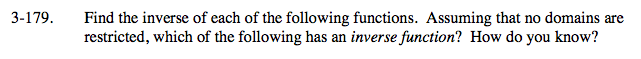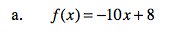Let f(x) = y.

Solve for x.

Switch the x and y.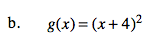Do not forget the ±. What will the graph of the inverse look like? Will there be exactly one output for each input?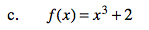To determine if the inverse is a function: Visualize! What does a cube root graph look like?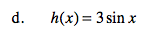If f(x) = sin(x)
Then f −1(x) = arcsin(x) = sin−1(x)
Note: cscx is the reciprocal of sinx, not the inverse!